# Problemsa) A 2000 kg car is travelling 50 miles per hour. Find the kinetic energy in Joules. b) The same car is lifted vertically upward and then dropped from rest. Find the height from which it is dropped if it strikes the ground at 50 miles per hour (neglect air resistance).

Solution:

a)

 KE =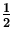mv 2 =(2 x 103  kg )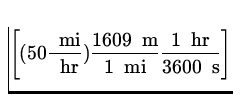2 = 4.99 x 105  J (10)
b)

 PEi = KEf mgh =mv 2h ==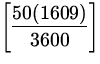2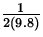= 25.5  m (11)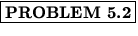An object of mass 1 kg travelling at 5.0 m/s enters a region of ice where the coefficient of kinetic friction is .10. Use the work energy theorem to find the distance the object travels before coming to rest.

Solution:The work energy theorem gives W =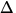KE . We have W = - fkd = -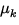Nd = -mgd andKE =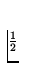mvf2 -mvi2 = -mvi2. Combining,

 -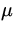mgd = -mvi2d =vi2 =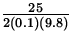= 13  m. (12)A 30 kg child enters the final section of a waterslide travelling at 2.0 m/s. The final section is 5.0 m long and has a vertical drop of 3.0 m. The force of friction opposing the child's motion is 50 N. Find a) the loss of potential energy, b) the work done by friction in the final section and c) the child's velocity at the end of the section (using energy considerations).

Solution:a)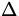PE = mg(hf - hi) = 30(9.8)(0 - 3) = - 882  J (13)
b)

 W = - fkx = - 50(5) = - 250  J (14)
c)

 Wnc =KE +PE- 250 =(30)(vf2) -(30)(2.0)2 - 882vf2 =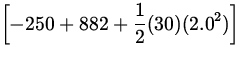vf = 6.8  m/s (15)A 2.0 kg wood block is on a level board and held against a spring of spring constant k=100 N/m which has been compressed .1 m. The block is released and pushed horizontally across the board. The coefficient of friction between the block and the board is= .20. Find a) the velocity of the block just as it leaves the spring and b) the distance the block travels after it leaves the spring.

Solution:

a)
The work energy theorem gives:
 Wnc =KE +PE - fkx = (mvf2 - 0) + (0 -kx 2) -mgx =mvf2 -kx 2vf2 =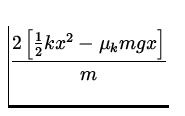=vf = 0.33  m/s. (16)
b)
The work energy theorem gives,
 -mgd = 0 -mvi2d =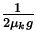vi2 =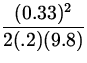= 0.028  m. (17)A man pushes a 100 kg box across a level floor at a constant speed of 2.0 m/s for 10 s. If the coefficient of friction between the box and the floor is= 0.20 , find the average power output by the man.

Solution: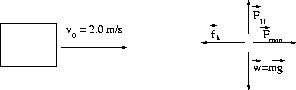Since the acceleration of the box is zero the force exerted by the man is obtained from F man - fk = 0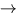F man = fk =mg . Then

 P == F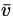=mg= .2(100)(9.8)(2) = 392  W. (18)